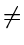Next: 4.1.2 Preorder, Inorder, Postorder Up: 4.1 Introduction Previous: 4.1 Introduction

## 4.1.1 Definitions

We shall first present some definitions and then introduce the Tree ADT.
• Tree:
1.
A single node is a tree and this node is the root of the tree.
2.
Suppose r is a node and T1, T2,..., Tk are trees with roots r1, r2,..., rk, respectively, then we can construct a new tree whose root is r and T1, T2,..., Tk are the subtrees of the root. The nodes r1, r2,..., rk are called the children of r.See Figure 4.1. Often, it is convenient to include among trees, the null tree, which is a tree with no nodes.

• Path

A sequence of nodes n1, n2,..., nk, such that ni is the parent of ni + 1 for i = 1, 2,..., k - 1. The length of a path is 1 less than the number of nodes on the path. Thus there is a path of length zero from a node to itself.

• Siblings

The children of a node are called siblings of each other.

• Ancestor and Descendent

If there is a path from node a to node b, then

a is called an ancestor of b

b is called a descendent of a

If ab, then a is a proper ancestor and b is a proper descendent.

• Subtree

A subtree of a tree is a node in that tree together with all its descendents.

• Height

The height of a node in a tree is the length of a longest path from the node to a leaf. The height of a tree is the height of its root.

• Depth

The depth of a node is the length of the unique path from the root to the node.

• Tree Traversal

This is a systematic way of ordering the nodes of a tree. There are three popular schemes (many other schemes are possible):

1.
Preorder
2.
Inorder
3.
Postorder

All these are recursive ways of listing or visiting all the nodes (each node exactly once)Next: 4.1.2 Preorder, Inorder, Postorder Up: 4.1 Introduction Previous: 4.1 Introduction
eEL,CSA_Dept,IISc,Bangalore